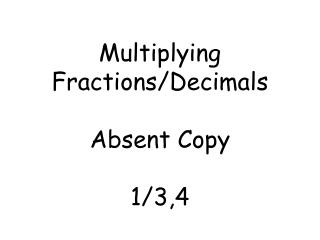# Multiplying Fractions/Decimals Absent Copy 1/3,4 - PowerPoint PPT PresentationDownload PresentationMultiplying Fractions/Decimals Absent Copy 1/3,4

Multiplying Fractions/Decimals Absent Copy 1/3,4Download Presentation## Multiplying Fractions/Decimals Absent Copy 1/3,4

- - - - - - - - - - - - - - - - - - - - - - - - - - - E N D - - - - - - - - - - - - - - - - - - - - - - - - - - -
##### Presentation Transcript

1. Multiplying Fractions/DecimalsAbsent Copy1/3,4

2. Example 1 • Multiply: write answer in simplest form. 3 • 7 4 9 3 • 7 = 21 4 • 9 36 Num. Denom. 21 36 7 • 3 2 • 2 • 3 • 3 7 • 3 2 • 2 •3 • 3 Solution • Do we need to get a common denom. when we multiply? • YES or NO • There are 2 ways to multiply. • 1. You can multiply straight across. • 2. You can cross reduce(only if you can) and then multiply across. • Can we reduce are answer? • YES or NO • What do we make before reducing the answer? • A T-chart 7 12

3. Example 2 • Multiply: write answer in simplest form. -2 ¾(½) -2 ¾ = -11 4 -11 • 1 = -11 4 2 8 -1 3/8 Solution • What do we do first before multiplying? We need to change the mixed fraction into an improper fraction. • There are 2 ways to multiply. • 1.You can multiply straight across. • 2.You can cross reduce (only if you can) and then multiply across. • Can we reduce are answer? • YES or NO • What question do we ask are selves. Hint: think about the 8. • How many times does 8 go into 11 and how many are left over. • What is the integer rule we use? • A neg. # multiplied by a pos. # the answer is always Neg. -1 3/8

4. Example 3 • Multiply: -0.06(9.3) -0.06 x9.3 018 + 0540 0558 0.558 Solution • How can we re-write this problem before multiplying? • Stack the numbers on top of each other before you multiply. • Do we need to line up the decimals when we multiply? YES or NO • After we multiply how many decimals places do we have to move in the answer? • We have to move the decimal 3 places. • Do we move the decimal left or right when making the final answer? • We move the decimal to the left from the back of the answer. • What is the integer rule we use? • Neg. # multiplied by a pos. # is always a neg. answer. -0.558

5. Example 4 • Multiply: -6.7 x -0.8 536 +000 536 5.36 Solution Solution • After we multiply how many decimals places do we have to move in the answer? • We have to move the decimal 2 places. • Do we move the decimal left or right when making the final answer? • We move the decimal to the left from the back of the answer. • What is the integer rule we use? • When multiplying a neg. # by a Neg. # the answer is always positive. 5.36